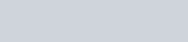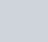If a two digit number is chosen at random, then the
Question:

If a two digit number is chosen at random, then the probability that the number chosen is a multiple of 3, is

(a) $\frac{3}{10}$

(b) $\frac{29}{100}$

(c) $\frac{1}{3}$

(d) $\frac{7}{25}$

Solution:

GIVEN: A two digit number is chosen at random

TO FIND: Probability that the number chosen is a multiple of 3

Total two digit numbers is 90

Two digit Numbers multiple of 3 are 12,15,18,21,24,27,30,33,36,39,42,45,48,51,54,57,60,63,66,69,72,75,78,81,84,87,90,93,96,99

Hence total two digit numbers multiple of 3 are 30

We know that PROBABILITY =Hence probability that the number chosen is a multiple of 3 is equal toHence the correct option is